客服热线：400-022-1280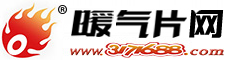18610894379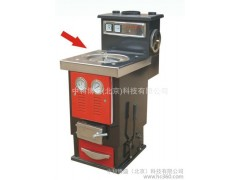•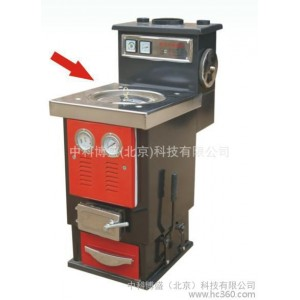••# 【中科帝火】 厂家热销无烟环保全自动炉民用采暖炉 燃煤锅炉

• 面议

≥1套

18610894379 01060256577
• 发货地  北京
• 供货总量  1000 套
• 品 牌  中科帝火
• 经营模式|制造商
• 注册资本|未填写
• 企业类型|企业单位（制造商）
• 主营产品|
• 公司地区|北京
• 公司荣誉|

• 暂无产品目录

 品牌中科帝火 有效期至长期有效 最后更新2017-10-26 05:12 浏览次数913

### 【中科帝火】 厂家热销无烟环保全自动炉民用采暖炉 燃煤锅炉

r    r        r            r        r        r            r            r            r        r        r            r            r            r        r    r
 r                rr                r r                r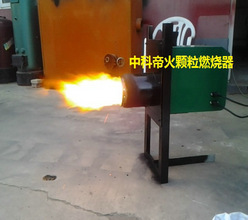r                r                r                    r                r                r                     生物质燃烧器r                r                r                    r                r r                r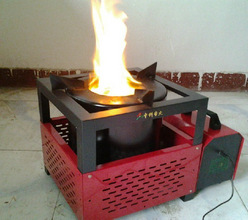r                r                r                    r                r                r                    生物质炊事炉r                r r                r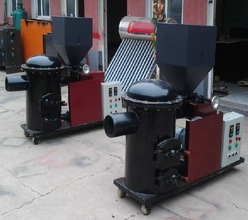r                r                r                    r                r                r                    生物质燃烧机r                r r                r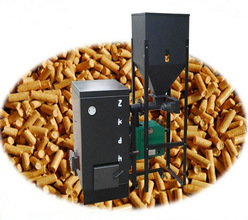r                r                r                    r                r                r                       生物质常压热水供暖炉r                r r                r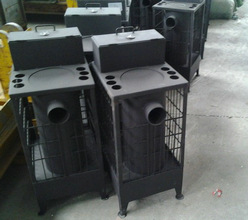r                r                r                    r                r                r                      生物质供暖炉r                r r                r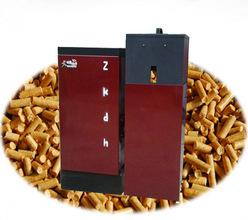r                r                r                    r                r                r                    生物质供暖锅炉r                r
r

r
r

r

r    温馨提示：r

r

r    产品属性及价格仅供参考，详情请来电或旺旺咨询！r

r

rr

r

r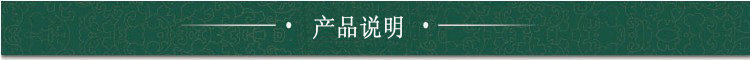r

r

r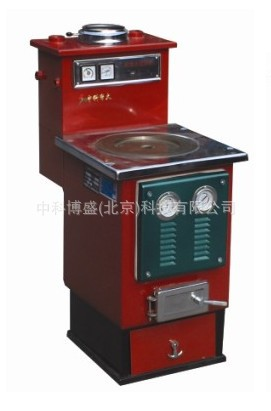rr

r

r    多燃料半气化炊暖炉：上火速度快     节能显著   低排高效r

r

r    中科帝火 自主专利产品，集炊事、采暖于一体、环保锅炉之优点，运用科学设计、研制的新一代环保、节能产品，领导了采暖、炊事炉具新潮流。
r   一、烧烟煤、秸秆压块 、烟筒不冒黑烟。通过炉体，炉口等多次合理配氧，使烟煤或秸秆压块等充分燃烧并达到节能和室内、室外无烟，无味、无焦油的效果。
r   二、炊事时，正压智能气化燃烧，火力猛，采暖时智能负压气化燃烧。烧水、做饭时火焰会随炊具移动而跟着移动且不熏炊具。
r   三、无可比拟的卫生、洁净燃烧，加煤时烟尘不出炉口、不上扬。
r   四、供暖或炊事时中途加煤可正常使用，不影响火力。r

r

r    五、实实在在的采暖输出功率，换热面积大，比同类型产品热效率提高30%。r

r

r    六、食品级10米不锈钢换热洗浴功能，进凉水出热水，为您一年四季炊、暖、浴等无忧使用。r

r

r    七、安全可靠，常压运行，正常使用无煤气中毒现象。r

r

r    八、操作简单，与原始炉、灶等点火、使用无两样，南北皆宜。r

r

r
r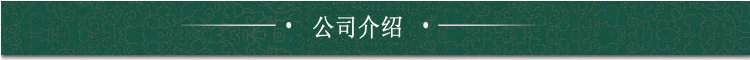r

r

r           我公司是专业从事新能源产品的研发与推广单位、拥有“中科帝火'自主品牌和自主知识产权的新能源系列环保锅炉、无烟囱环保锅炉、浴池专用锅炉、生物质锅炉、数控环保锅炉、供暖机器人、半气化民用采暖炉、燃煤气化采暖炉、太阳能系列、电磁太阳能光电互补采暖、光波采暖热水炉、光波太阳能供暖系统、24小时太阳能集中供热水系统、燃煤净化设备、地暖专用环保锅炉、地暖水垢处理仪、电磁热水采暖、纳米光波热水采暖炉、超强对流散热器、温室热风炉、自动加料采暖炉、旋动燃烧气化炉、节柴 省柴炉、新型散热器等产品专业研发、加工的有限责任公司。设有四个新产品、新技术研发基地、六个新产品生产加工基地、并设有中科帝火新能源产品（辽宁锦州）发展基地、中科帝火（北京门头沟区）新能源产品发展基地、中科帝火（北京大兴区）新能源产品发展基地、中科帝火（甘肃酒泉地区）新能源产品办事处、中科帝火（青海格尔木地区）新能源产品办事处等。中科帝火（山东）新能源产品基地等。总部设在北京大兴区西红门工业园,我公司本着完整、严格、规范的按照ISO9000国际质量管理体系执行。以诚信加实力的产品技术、质量并获得业界的一致认可。欢迎各界新老朋友莅临指导和业务洽谈。
r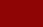r公司名 经营模式 中科博盛（北京）新能源科技有限公司 制造商 未填写 2009 北京 企业单位 (制造商) 已缴纳 0.00 元 材质分类 / 钢制散热器系列  ,© xiaohei
0相关评论